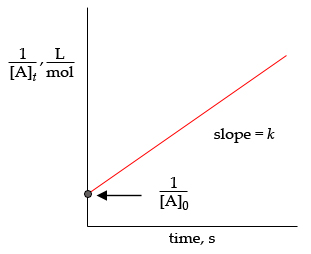# 1 L [Al mol slope = k [Alo time, s

Question

For a second-order reaction, [A]products, the rate of the reaction is given as rate= k[A]2, where k is the rate constant and [A] is the concentration of reactant A. The integrated rate law for second-order reactions is 1[A]t=kt+1[A]0, where [A]t is the concentration of reactant Aat time tk is the rate constant, and [A]0 is the initial concentration of reactant A. This equation is of the type y=mx+b. Therefore, the plot of 1[A]t versus time is always a straight line with a slope k and a y intercept 1[A]0.

Consider the second-order reaction: 2HI(g)H2(g)+I2(g)Use the simulation to find the initial concentration [HI]0 and the rate constant k for the reaction. What will be the concentration of HI after t = 3.92×1010 s ([HI]t) for a reaction starting under the condition in the simulation?help_outlineImage Transcriptionclose1 L [Al mol slope = k [Alo time, s fullscreen

## Expert Answer

### Want to see this answer and more?

Experts are waiting 24/7 to provide step-by-step solutions in as fast as 30 minutes!*

*Response times may vary by subject and question complexity. Median response time is 34 minutes for paid subscribers and may be longer for promotional offers.
Tagged in
Science
Chemistry

### Chemical Kinetics© 2021 bartleby. All Rights Reserved.